# Quantitative analysis for effects of structural stiffness on vibration characteristics of machine tool feed system

## Niancong Liu1

1School of Mechanical Engineering, Southwest Jiaotong University, Chengdu, 610031, China

1School of Nuclear Technology and Automation Engineering, Chengdu University of Technology, Chengdu, 610059, China

Journal of Vibroengineering, Vol. 22, Issue 2, 2020, p. 241-251. https://doi.org/10.21595/jve.2019.20332
Received 24 October 2018; received in revised form 19 August 2019; accepted 5 October 2019; published 31 March 2020

Copyright © 2020 Niancong Liu. This is an open access article distributed under the Creative Commons Attribution License, which permits unrestricted use, distribution, and reproduction in any medium, provided the original work is properly cited.
Views 203
Abstract.

The vibration of the feed system remains a long-standing challenge in a machine. Toward this objective, the effects of bearing stiffness, nut rigidity, coupling stiffness, screw length, screw speed and radial stiffness of worktable on the torsional vibration and axial vibration of the table are explored. In this paper, a mathematical model of multi-degree-of-freedom coupling torsional and axial vibration considering various factors is established by the second Lagrange equation, and simulation analysis and experimental verification are carried out. The effects of the variable lead and variable torsional stiffness of the nut on the torsional vibration of the workbench are analyzed. Results show that nut stiffness is the main factor affecting the vibration of the workbench. As the workbench mass, motor speed, screw length and lead increase, the axial vibration of the workbench increases. In the case of lead change, as the axial stiffness of the nut increases, the effect on the torsional vibration of the workbench is reduced. Under the variable torsional stiffness of the nut, with the increase of the lead, the influence of the lead on the torsional vibration of the workbench will increase. The experimental results provide a reference for further research and provide a theoretical basis for vibration reduction and structural optimization.

Highlights
• This paper discusses the influence of the stiffness of bearings, nuts, couplings and other parts on the torsional and axial vibration of the workbench.
• The dynamic model of multi degree-of-freedom coupling torsional and axial vibration of feed system is established in this paper.
• This paper provides a theoretical basis for the vibration reduction and structural optimization of the feed system.

Keywords: feed system, multiple degree-of-freedom, Lagrange equation, axial vibration, torsional vibration, influence factor.

#### 1. Introduction

The complicated ball screw feed system is the main component of the CNC machine tools . The transmission mode of the feed system parts largely affects the vibration characteristics of machine tools, thus affecting the quality and precision of machining. Therefore, in order to improve the machining quality and precision, the study of vibration characteristics of feed system and the key factors affecting feed system has become a hot topic for most researchers [1-5]. Ansoategui et al. adopted Newton formulation to establish the lumped-parameter dynamic structural model of the feed system in modal coordinates, and compared it with the model in natural coordinates. Although the validity of the model in modal coordinates was proved, the study only analyzed the related vibration characteristics under torsional modes . Zou et al. studied the influence of screw-nut stiffness on the vibration characteristics of the spindle system in the transmission direction. Based on the equivalent dynamic equation and the D’Alembert’s principle, the varying-coefficients model of feed system was established, but the influence of coupling characteristics was neglected . Vicente et al. established a mathematical model, which is coupled with torsional and axial system by using Ritz series method, to analyze the influence of lead and workbench position . The coupled and non-coupled model frequencies are analyzed and compared, but the influence is neglected. Meanwhile, the torsion of the workbench during modeling did not reach a certain effect trend. Weng et al. used ANSYS to establish a finite element model considering joints, and analyzed the effects of various joints stiffness on longitudinal vibration dynamic performance through modal analysis and harmonic response analysis . However, they did not establish an effective mathematical model that was only analyzed by software, which in turn comprehensively and quantitatively analyzed various factors. To study the dynamic characteristics of one-axle stage under heavy load, an equivalent finite element model of one-axle stage under heavy load was proposed, but the boundary conditions of bolted connections were ignored in the modeling .

Therefore, to reduce the vibration in the feed, it is important to perform a full study taking into account all the possible factors affecting the vibration of the system. In this paper, an analytical dynamic model was developed, which takes into account the influence of the stiffness of the joint such as the coupling comprehensively, and the influence law of each factor on the torsional-axial vibration of the worktable of the feed system is analyzed quantitatively.

#### 2. Mathematical model of feed system

The feed system contains ten different parts mainly, the name and the position of each part are shown in Fig. 1.

Fig. 1. The structure of the feed system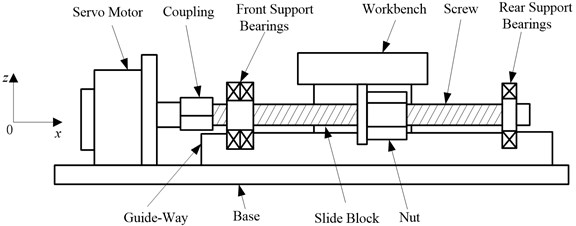The structure of the feed system is simplified as follows in order to establish a better mechanical model:

(1) The motor shaft is short and thick, and its rigidity is large, so it is considered as a rigid body.

(2) The coupling is simplified into an equivalent torsional spring consisting of torsional stiffness and torsional damping.

(3) Each joint is simplified into a damper consisting of the axial stiffness and axial damping along the $x$ direction and torsional stiffness and torsional damping around the $x$ direction.

(4) The workbench-guideway joint is simplified into a damper composed of damping and stiffness along the $z$ direction.

(5) The screw is considered as an elastic body.

(6) Regard the workbench as a centralized mass.

The feed system model can be simplified as Fig. 2.

As shown in Fig. 2, $\theta$ and ${\theta }_{1}$ represent the input angular displacement of the motor shaft rotating around the x direction and the input angular displacement of the screw beside the motor, respectively; ${J}_{2}$, ${J}_{1}$, and ${J}_{3}$ stand for the moment of inertia of coupling, the moment of inertia of motor and the moment of inertia of workbench around the $x$ direction, respectively; ${c}_{m}$ is the viscous damping of the motor around the $x$ direction; ${k}_{1}$ and ${c}_{1}$ represent torsional stiffness and the damping of coupling; ${k}_{2}$, ${k}_{5}$ and ${c}_{2}$, ${c}_{5}$ stand for the axial stiffness and the axial damping of two screw-bearing joints along the $x$ direction; ${k}_{3}$, ${k}_{6}$ and ${c}_{3}$, ${c}_{6}$ represent the torsional stiffness and the torsional damping of two screw-bearing joints around the $x$ direction; ${k}_{8}$ and ${k}_{9}$, ${c}_{8}$ and ${c}_{9}$ stand for the axial stiffness along the $x$ direction and the torsional stiffness around the $x$ direction of the screw-nut joint, the axial damping along the $x$ direction and the torsional damping around the $x$ direction; ${k}_{11}$, ${k}_{12}$ and ${c}_{11}$, ${c}_{12}$ represent the radial stiffness and the radial damping of two workbench-guideway joints along the $z$ direction; ${c}_{t}$ is the axial viscous damping between workbench and guideway along the $x$ direction; $m$ is the mass of workbench; $u\left(x,t\right)$ and $v\left(x,t\right)$ represent the axial vibration displacement along the $x$ direction and the torsional vibration displacement around the $x$ direction of the screw, respectively; $s\left(t\right)$ and $\phi \left(t\right)$ are the axial displacement and the torsional displacement of the workbench; ${L}_{1}$ is length of screw at workbench.

The second kind of Lagrange equation is expressed as:

(1)
$\frac{d}{dt}\left(\frac{\delta L}{\delta {\stackrel{˙}{q}}_{i}}\right)-\frac{\delta L}{\delta {q}_{i}}+\frac{\delta D}{\delta {\stackrel{˙}{q}}_{i}}=Q,$

with $L=T-V$, where $T$, and $V$ are kinetic energy, and potential energy. $L$, $D$, $Q$ and ${q}_{i}$ represent difference between kinetic energy and potential energy, damping energy, generalized force, and generalized coordinate of the system.

Kinetic energy of the system includes the kinetic energy of the workbench, the axial and the torsional vibration kinetic energy of the screw, etc., as shown in Eq. (2):

(2)

where $J$, $A$, $\rho$ and $d$ are the polar moment of inertia, the cross-sectional area, the density and the diameter of screw; $b$ is the length of workbench along the $y$ direction.

Fig. 2. The simplified model of the feed system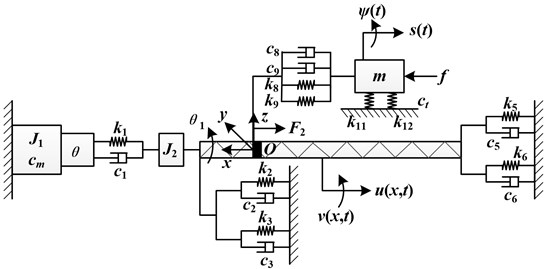The potential energy of the system includes the deformation energy of the screw and the elastic potential energy of each joint, as shown in Eq. (3):

(3)
$V=\frac{1}{2}{k}_{1}{\left[{\theta }_{1}-\theta +v\left(0,t\right)\right]}^{2}+\frac{1}{2}{k}_{2}{u}^{2}\left(0,t\right)+\frac{1}{2}{k}_{6}{v}^{2}\left(L,t\right)+\frac{1}{2}{k}_{5}{u}^{2}\left(L,t\right)$

where $G$, $E$, $l$ and $L$ are the modulus of Poisson, Young’s modulus, lead and length of the screw, respectively.

The damping energy of the system includes energy consumption of the structural damping of the screw and damping at joints, as shown in Eq. (4):

(4)
$D=\frac{1}{2}{c}_{8}{\left(\stackrel{˙}{s}-l\stackrel{˙}{\varphi }-\frac{\partial u\left(x,t\right)}{\partial t}-l{\stackrel{˙}{\theta }}_{1}\right)}^{2}+\frac{1}{2}{c}_{2}{\left(\frac{\partial u\left(0,t\right)}{\partial t}\right)}^{2}+\frac{1}{2}{c}_{5}{\left(\frac{\partial u\left(L,t\right)}{\partial t}\right)}^{2}$

where ${c}_{t2}$ is the torsional stiffness of workbench.

The virtual work done by the external force of the system is described as:

(5)
$\left\{\begin{array}{l}\partial W=\left(F+f\right)\partial x,\\ f={f}_{1}sign\left(\stackrel{˙}{s}\right)+{f}_{2}\stackrel{˙}{s},\end{array}\right\$

where $F$ is the nut preload, which is calculated at 10 % of the maximum dynamic load; $f$ is the resistance of the workbench; ${f}_{1}$ and ${f}_{2}$ are Coulomb friction coefficient and static friction coefficient of the workbench.

Based on the hypothetical mode method, the torsional vibration displacement and the axial vibration displacement of the screw can be divided into two functions expressed by displacement and time [11, 12]:

(6)
$\left\{\begin{array}{l}u\left(x,t\right)=\sum _{i=1}^{N}\mathrm{c}\mathrm{o}\mathrm{s}\left(\frac{\left(i-1\right)\pi x}{L}\right){q}_{u}\left(t\right),\\ v\left(x,t\right)=\sum _{i=1}^{N}\mathrm{c}\mathrm{o}\mathrm{s}\left(\frac{\left(i-1\right)\pi x}{L}\right){q}_{v}\left(t\right),\end{array}\right\$

where ${q}_{u}\left(t\right)$ and ${q}_{v}\left(t\right)$ are the axial independent coordinate and the torsional independent coordinate of the screw respectively, 3.

Solving Eqs. (1), (2), and (6), the dynamic equation of the feed system can be obtained as:

(7)
$M\left[{\stackrel{¨}{q}}_{i}\right]+C\left[{\stackrel{˙}{q}}_{i}\right]+K\left[{q}_{i}\right]=F\left(t\right),$

where $M$, $C$, and $K$ are the inertia, damping, and stiffness matrices, respectively (see Appendix); ${q}_{i}$ is the generalized coordinates; $F\left(t\right)$ is the generalized forces.

From the matrix determinant, it can be concluded that the main factors affecting the dynamic performance of the system are the mass of the workbench, the moment of inertia of the rotating parts, the stiffness and damping of the components, and the position of the workbench and so on.

#### 3. Experimental verification of dynamic model

The ball screw feed system of high-speed precision CNC lathe (Model CK6136S, Zhejiang Kaida Machine Tool Group Co., Ltd., China) is selected to verify the reliability of the dynamic model. The axial dynamic response characteristics of the feed system are tested in experiments. The dynamic equation of the axial vibration is decoupled and solved by Matlab. The main simulation parameters of the feed system obtained from measurements and calculations are shown in Table 1 .

Table 1. Main calculating parameters of feed system

 Parameter Value Parameter Value $\rho$ / (kg∙m-3) 7.9×103 ${k}_{1}$ / (N∙m-1) 4.2×105 /m2 8.1×10-4 ${k}_{2}$, ${k}_{5}$ / (N∙m-1) 5.6×105 $L$ / m 1.5 ${k}_{3}$, ${k}_{6}$ / (N∙m.rad-1) 6.9×104 ${L}_{1}$ / m 0.45 ${k}_{8}$ / (N∙m-1) 1.0×107 $l$ / m 6×10-3 ${k}_{9}$ / (N∙m.rad-1) 5.9×104 $r$ / m 3.2×10-2 $E$ / GPa 206 $m$ / kg 136 $G$ / GPa 78.6 $J$ / (kg∙m2) 1.2×10-3 $b$ / m 0.83 ${J}_{1}$/ (kg∙m2) 2.1×10-3 ${k}_{11}$, ${k}_{12}$ / (N∙m-1) 5.5×109 ${J}_{2}$ / (kg∙m2) 1.3×10-4

In order to simplify the analysis, the damping is 0. In the simulation, the motor torque is used as input and the axial acceleration of workbench is used as output. The spectrum analysis results are shown in Fig. 3.

The test principle of the experiment is shown in Fig. 4. The vibration test device mainly contains DH5922N dynamic signal test and analysis system, and DH311 piezoelectric acceleration sensor and so on. $X$-axis sensitivity of DH311 sensor is 0.97 pc/m∙s-2, the range is 0-5000 ms-2 and the frequency response is 0-5 kHz. In the $x$-axis feed experiment, the spindle of NC lathe is stationary, the feed displacement is 25 mm, the feed speed is 300 mm/min, and the acceleration is 0.5 g. The axial acceleration signal of the workbench is collected, intercepted in condition of uniform motion and analyzed in frequency domain.

Fig. 3. Simulated result of frequency response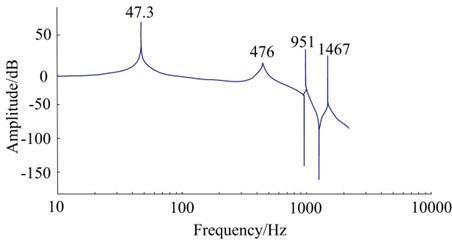Fig. 4. Testing principle of the experiment table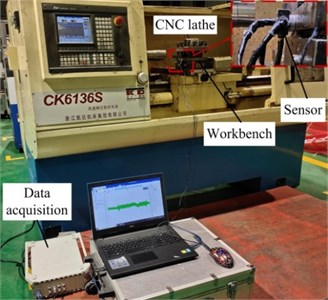Fig. 5. Spectrum analysis of dynamic characteristics test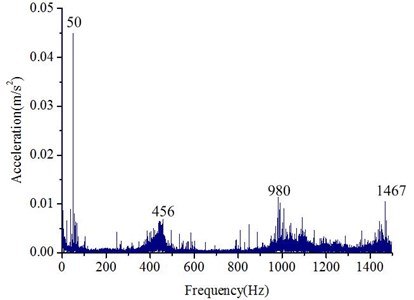From Fig. 3 and Fig. 5, there are four characteristic frequencies within 0-2 kHz, and they are basically consistent. The comparison between theoretical and measured characteristic frequencies is shown in Table 2. Because of the simplification of geometric model, the error of finite element calculation and test, there are differences between the theoretical characteristic frequency and the experimental characteristic frequency. However, the corresponding frequency errors are less than 6 %. Compared with reference , although Lagrange equation is used to establish the dynamic model of feed system, the error is more than 6.3 %. It can be seen that the modeling method can accurately reflect the dynamic characteristics of the ball screw feed system.

Table 2. Comparison of characteristic frequency

 Order $i$ Simulation results / Hz Experimental results / Hz Error $\sigma$ / % 1 47.3 50 5.7 2 476 456 4.2 3 951 980 3.0 4 1419 1467 3.3

#### 4. Vibration characteristic analysis

The parameters are adjusted by integral multiples based on dynamic model as for that the torsional vibration around the $x$ direction and axial vibration along the $x$ direction of the workbench have a different influence on machining accuracy. Meanwhile, the influence of the characteristic parameters on the acceleration range of the coupled torsional and axial vibration of the workbench is analyzed. In order to make the conclusion more universal, the product specifications and the calculation method of relevant literature [15, 16] are consulted, and the initial parameters of the feed system commonly used in the numerical control machine tools for analysis are determined, as shown in Table 3.

Table 3. Parameter values used in the model

 Parameter Value Unit ${k}_{1}$ 416666 N/rad ${k}_{2}$, ${k}_{5}$ 50000 N/m ${k}_{3}$, ${k}_{6}$ 10 rad/m ${k}_{8}$ 6.08×108 N/m ${k}_{9}$ 385 N/rad ${k}_{11}$, ${k}_{12}$ 4.56×109 N/m $b$ 0.25 m

When the workbench position $x$ is a fixed, the workbench is excited by a force. When the stiffness is increased from the original value to 5 times, the acceleration range of the torsional vibration of the workbench is shown in Table 4. As the other stiffness increases, it is equal to the acceleration rang of the axial vibration of the workbench (Table 5). In Table 4 and 5, the rate of decline indicates the degree of decrease in the acceleration range of the vibration of the workbench when the parameter is increased from the original value to 5 times.

As shown in Table 4, with the increase in the torsional stiffness of the screw-bearing joint, coupling, screw-nut joint and the radial stiffness of the workbench-guideway joint, the torsional vibration acceleration of the workbench is decreased. In addition, the rate of descent in Table 4 indicates that the radial stiffness of the workbench-guideway joint and the torsional stiffness of the screw-nut joint are the main factors affecting the torsional vibration of the workbench. However, the torsional stiffness of the bearing affects is least. The reason is that the radial stiffness of the workbench-guideway joint and the torsional stiffness of the screw-nut joint are quite large, and the torsional stiffness of the nut in system is strongly coupled. For the torsional stiffness of the bearing, it is less than other torsional stiffness and the coupling of the bearing is smaller than the nut. The coupling has a large effect on the torsional vibration of the workbench, which is relatively small compared with the radial stiffness of the workbench-guideway joint and the torsional stiffness of screw-nut joint. This is because the coupling that is a direct component of the transmitted torsional motion.

As shown in Table 5, with the increase of the axial and torsional stiffness of the screw-nut joint, the torsional stiffness of the bearing joint and the axial stiffness of the bearing joint, the axial vibration amplitude of the table decreases gradually.

Table 4. The influence of the stiffness on the acceleration range of the torsional vibration of the workbench

 Acceleration range (rad/s2)-(E-13) Original 2 times 3 times 4 times 5 times Rate of decline (%) ${k}_{9}$ 7.1167 5.7514 4.8177 4.1394 3.6240 49.07 ${k}_{11}$, ${k}_{12}$ 7.1167 3.5592 2.3729 1.7798 1.4239 80.00 ${k}_{3}$, ${k}_{6}$ 7.1167 7.0917 7.0670 7.0427 7.0187 1.38 ${k}_{1}$ 7.1167 6.4871 5.9598 5.5118 5.1263 27.97

Table 5. The influence of the stiffness on the acceleration range of the axial vibration of the workbench

 Acceleration range (m/s2)-(E-8) Original 2 times 3 times 4 times 5 times Rate of decline (%) ${k}_{8}$ 9.4145 4.7158 3.1458 2.3600 1.8884 79.94 ${k}_{9}$ 9.4145 9.4076 9.4007 9.3938 9.3870 2.93 ${k}_{3}$, ${k}_{6}$ 9.4145 9.4123 9.4103 9.4084 9.4066 0.08 ${k}_{2}$, ${k}_{5}$ 9.4145 9.3970 9.3864 9.3804 9.3775 0.04

It can be seen from the decreasing rate of Table 5 that among the factors affecting the axial vibration of the workbench, the axial stiffness of the screw-nut joint has the greatest influence on the axial vibration of the workbench, and the descending rate is also largest and is 79.94 %, which is due to the strong coupling of the axial stiffness of the nut in the system and the direct connection with the worktable. The torsional stiffness of the screw-bearing joint has the least effect on the axial vibration of the workbench for three reasons. Firstly, the value of torsional stiffness of the screw-bearing joint is very small. Second, its coupling is relatively small compared with the nut. Finally, the coupling is not directly connected to the workbench. Compared the descent rates Table 4 and Table 5, we can find that, for same joint, the influence of the torsional stiffness on the axial vibration of workbench is significantly less than the axial stiffness of the axial vibration, such as the torsional stiffness and the axial stiffness of the screw-bearing joint and the screw-nut joint.

When the mass is reduced to a certain ratio, the acceleration range of the torsional vibration and the axial vibration of the workbench are shown in Table 6.

Table 6. The influence of the workbench mass on the acceleration range of the torsional vibration and the axial vibration of the workbench

 Mass Original 1/2 times 1/3 times 1/4 times 1/5 times Rate of decline (%) Torsional (rad/s2)-(E-13) 7.1167 6.9833 6.8719 6.7712 6.6869 6.09 Axial (m/s2)-(E-8) 9.4145 9.2437 9.1063 8.9873 8.8790 5.69

Table 6 shows that the torsional vibration and the axial vibration of the workbench are reduced with the decrease of the mass of the workbench. The reason for this is that, the reduce of the mass of the workbench making the moment of inertia reduced, as a result, the inertia force is reduced. Therefore, the vibration decreases.

When the length of the screw increases from 1 m to 2 m, the effect of axial stiffness of the nut on the acceleration range of the axial vibration of the workbench is shown in Table 7.

As shown in Table 7, as the length of the screw increases, the axial stiffness of the nut will gradually decrease the axial vibration of the workbench. At the same time, the influence of the length of the screw on the axial vibration of the workbench is less than the axial stiffness of the nut thorough analysis.

Table 7. The influence of the axial stiffness of the nut on the acceleration range of the axial vibration of the workbench under different screw’s length

 Acceleration range (m/s2)-(E-8) 1 time 2 times 3 times 4 times 5 times Rate of decline (%) Length (1 m) 9.4145 4.7158 3.1458 2.3600 1.8884 79.9435 Length (2 m) 9.4464 4.7308 3.1555 2.3673 1.8942 79.9416

The influence of the torsional stiffness of the screw-nut joint on the torsional vibration of the workbench under variable lead is shown in Table 8. In Table 8, rate of decline represents that under variable lead, when the torsional stiffness of the nut is increased by 5 times from the original value, the degree of the torsional vibration is reduced by the quantitative acceleration of the workbench. The increase rate indicates the degree to which the torsional amplitude of the workbench increases as the lead increases from 0.005 m to 0.01 m under the variable torsional stiffness of the nut.

Table 8. The influence of the torsional stiffness of the nut on the acceleration range of the torsional vibration of the workbench under different screw’s lead

Table 8 shows that after increasing the lead, the torsional stiffness of the nut will have a reduced effect on the torsional vibration of the workbench, and conversely, after increasing the torsional stiffness of the nut, the influence of the lead on the torsional vibration of the workbench will increase.

Fig. 6. The influence of the motor speed on the axial vibration of the workbench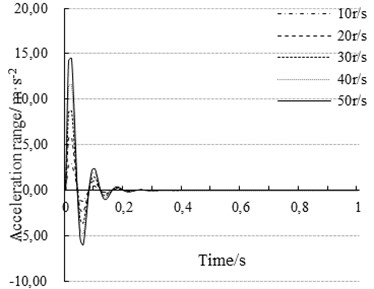When considering that the workbench position $x$ is not fixed, take $\theta =\omega t$, where $\omega$ is the motor speed. When the motor speed increased from 10 r/s to 50 r/s, the effect of motor speed on the acceleration range of the axial vibration of the workbench is shown in Fig. 6. It shows that with the increase of the motor speed, the axial vibration of the workbench will gradually increase.

#### 5. Conclusions

1. An axial mathematical model of multi-degree-of-freedom coupling torsional and axial vibration is proposed, which takes into account various factors. The simulation analysis and experimental verification of the feed system are carried out. The results show that the model has obvious advantages over the previous dynamic models. The error of characteristic frequency is the smallest, all less than 5 %, which shows that the model coincidence is good. Based on the dynamic model, the effects of several factors such as bearing stiffness, nut stiffness and coupling stiffness on torsional and axial vibration are analyzed quantitatively.

Based on the dynamic model, the effects of several factors such as bearing stiffness, nut stiffness and coupling stiffness on torsional and axial vibration are analyzed.

2. With the increase of the torsional stiffness of the screw-nut joint, the torsional stiffness of the screw-bearing joint, the radial stiffness of the workbench-guideway joint and the torsional stiffness of the coupling, the torsional vibration of the workbench decreases gradually. Among the four stiffness, the radial stiffness has the greatest influence, the torsional stiffness of the screw-nut joint is secondary, and the screw-bearing joint has the smallest torsional stiffness.

3. With the increase of the axial stiffness of the screw-nut joint, the axial stiffness of the screw-bearing joint, and the torsional stiffness of the coupling, the axial vibration of the workbench decreases gradually. However, the increase of mass, screw length and lead leads to the increase of axial vibration of the table, and the axial stiffness of the screw-nut joint has the largest influence.

4. Under the condition of variable lead, with the increase of the torsional stiffness of the screw-nut joint, the influence of the torsional stiffness on the torsional vibration of the workbench is decreased. The influence of the lead on the torsional vibration of the workbench will increase as the lead increases when the torsional stiffness of the screw-nut joint is changed.

5. When considering that the workbench position $x$ is not fixed, as the motor speed increase, the axial vibration of the workbench increases.

#### Acknowledgements

This study is supported by the National Natural Science Foundation of China (Grant No. 51575457), Sichuan Science and Technology Program (Grant No. 2019YFG0358), and the research project of Chengdu Science and Technology Bureau (Grant No. 2015-NY02-00285-NC).

1. Wang W., Zhou Y. X., Wang H., Li C. Y., Zhang Y. M. Vibration analysis of a coupled feed system with nonlinear kinematic joints. Mechanism and Machine Theory, Vol. 134, 2019, p. 562-581. [Publisher]
2. Lantz M. A., Cherubini G., Pantazi A., Jelitto J. Servo-patter design and track-following control for nanometer head positioning on flexible tape media. IEEE Transactions on Control System Technology, Vol. 20, Issue 2, 2012, p. 369-381. [Publisher]
3. Cheng Q., Qi B. B., Liu Z. F., Zhang C. X., Xue D. Y. An accuracy degradation analysis of ball screw mechanism considering time-varying motion and loading working conditions. Mechanism and Machine Theory, Vol. 134, 2019, p. 1-23. [Publisher]
4. Msukwa M. R., Uchiyama N. Design and experimental verification of adaptive sliding mode control for precision motion and energy saving in feed drive systems. IEEE Access, Vol. 7, 2019, p. 20178-20186. [Publisher]
5. Liu L. L., Liu H. Z., Wu Z. Y., Yuan D. N. Research on stability of feed servo system of machine tool at low speed. Journal of Vibration and Shock, Vol. 29, Issue 5, 2010, p. 187-190. [Search CrossRef]
6. Ansoategui I., Campa F. J. Mechatronics of a ball screw drive using an N degrees of freedom dynamic model. International Journal of Advanced Manufacturing Technology, Vol. 93, Issues 1-4, 2017, p. 1307-1318. [Publisher]
7. Zou C. F., Zhang H. J., Lu D., Zhang J., Zhao W. H. Effect of the screw-nut joint stiffness on the position-dependent dynamics of a vertical ball screw feed system without counterweight. Proceedings of the Institution of Mechanical Engineers Part C-Journal of Mechanical Engineering Science, Vol. 232, Issue 15, 2018, p. 2599-2609. [Publisher]
8. Vicente D. A., Hecker R. L., Villegas F. J., Flores G. M. Modeling and vibration mode analysis of a ball screw drive. The International Journal of Advanced Manufacturing Technology, Vol. 58, Issues 1-4, 2012, p. 257-265. [Publisher]
9. Weng K. K., Cheng Y., Xia L. L., Li K. The dynamic characteristics analysis of feeding system of a vertical machining center based on conjoint interfaces. Machinery Design and Manufacture, Vol. 3, 2012, p. 130-132. [Search CrossRef]
10. Jang W. Y., Park M. Y., Kim J. H., Kang E. G., Jeong J. I. Analysis of vibration characteristics of one-axis heavy duty stages. Journal of Mechanical Science and Technology, Vol. 31, Issue 12, 2017, p. 5721-5727. [Publisher]
11. Zhang H. R. Dynamic Analysis of Machine Tool Feed System. PhD Thesis, Jilin University, Changchun, 2009, (in Chinese). [Search CrossRef]
12. Yang X. J., Zhao W. H., Liu H. Coupled vibration mode analysis of a ball screw drive system. Machinery Design and Manufacture, Vol. 10, 2012, p. 259-261. [Search CrossRef]
13. Zhu J. M., Zhang T. C., Li X. R. Dynamic characteristic analysis of ball screw feed system based on stiffness characteristic of mechanical joints. Journal of Mechanical Engineering, Vol. 51, Issue 17, 2015, p. 72-82, (in Chinese). [Publisher]
14. Lu H., Fan W., Zhang X. B., Zhang Y., Wang S., Duan M. Dynamic characteristics analysis and test of dual-driving feed system driven by center of gravity. Mathematical Problems in Engineering, Vol. 2018, 2018, p. 9490826. [Publisher]
15. Gong Z. C., Niu W. T., Li J. J., Sai Y. X. Dynamic characteristics simulation and performance optimization of the feed system based on the electromechanical coupling. Journal of Machine Design, Vol. 35, Issue 9, 2018, p. 8-16. [Search CrossRef]
16. Wang M., Le B. B., Pei E. Y. Contact stiffness modeling and analysis of linear ball guides based on Hertz contact theory. Journal of Beijing University of Technology, Vol. 41, Issue 8, 2015, p. 1128-1132. [Search CrossRef]

#### Cited By

 Journal of Intelligent & Fuzzy Systems Wen-Hsiang Hsieh, Yi-Syun Chen, Shang-Teh Wu 2021 Industrial Lubrication and Tribology Feng Gao, Pan-Pan Li, Yan Li 2021 Journal of Vibroengineering Chao Qiu, Xingzhou Chen, Yanbo Hui, Tahir Abdul Rahman Siddiquee 2020